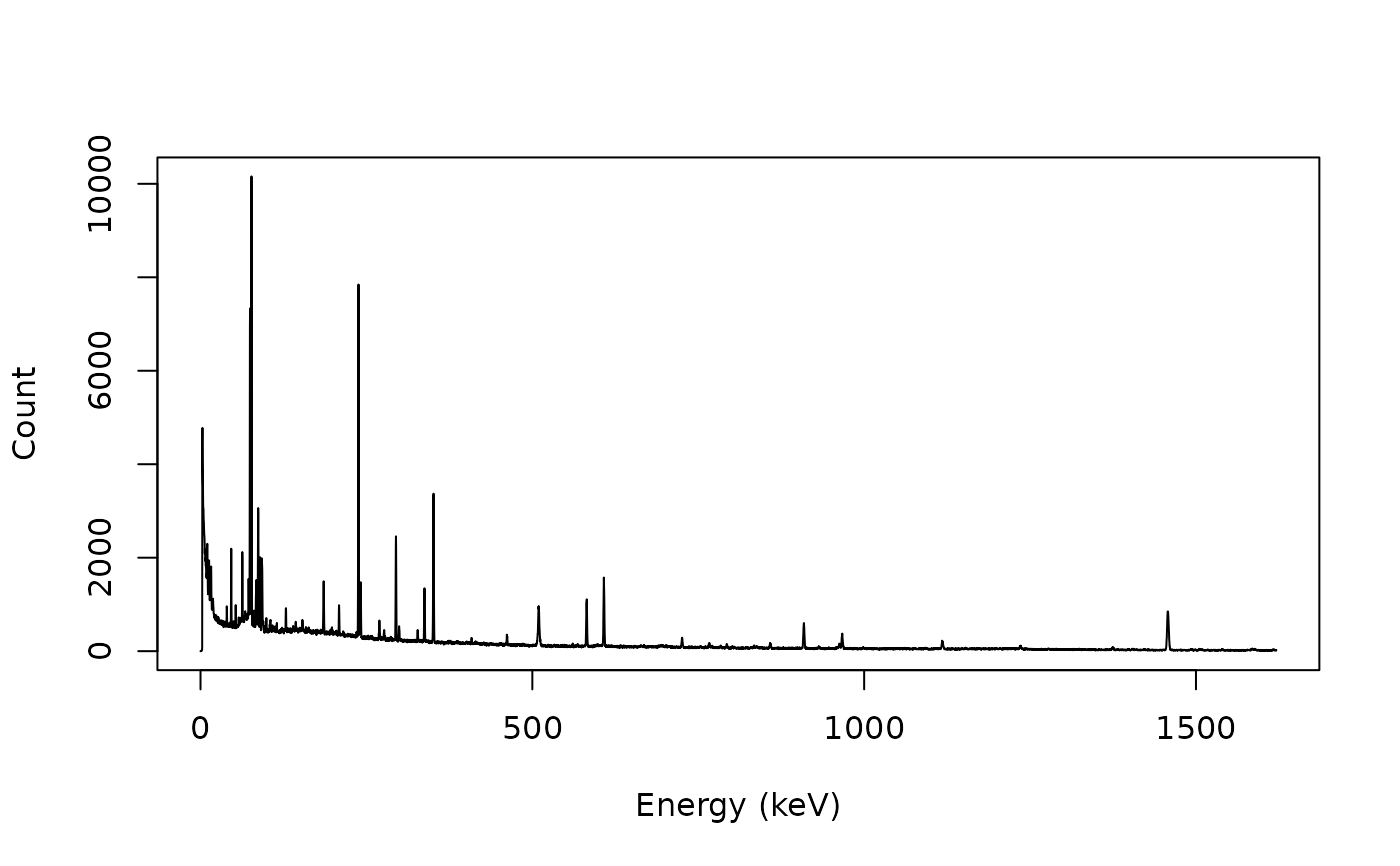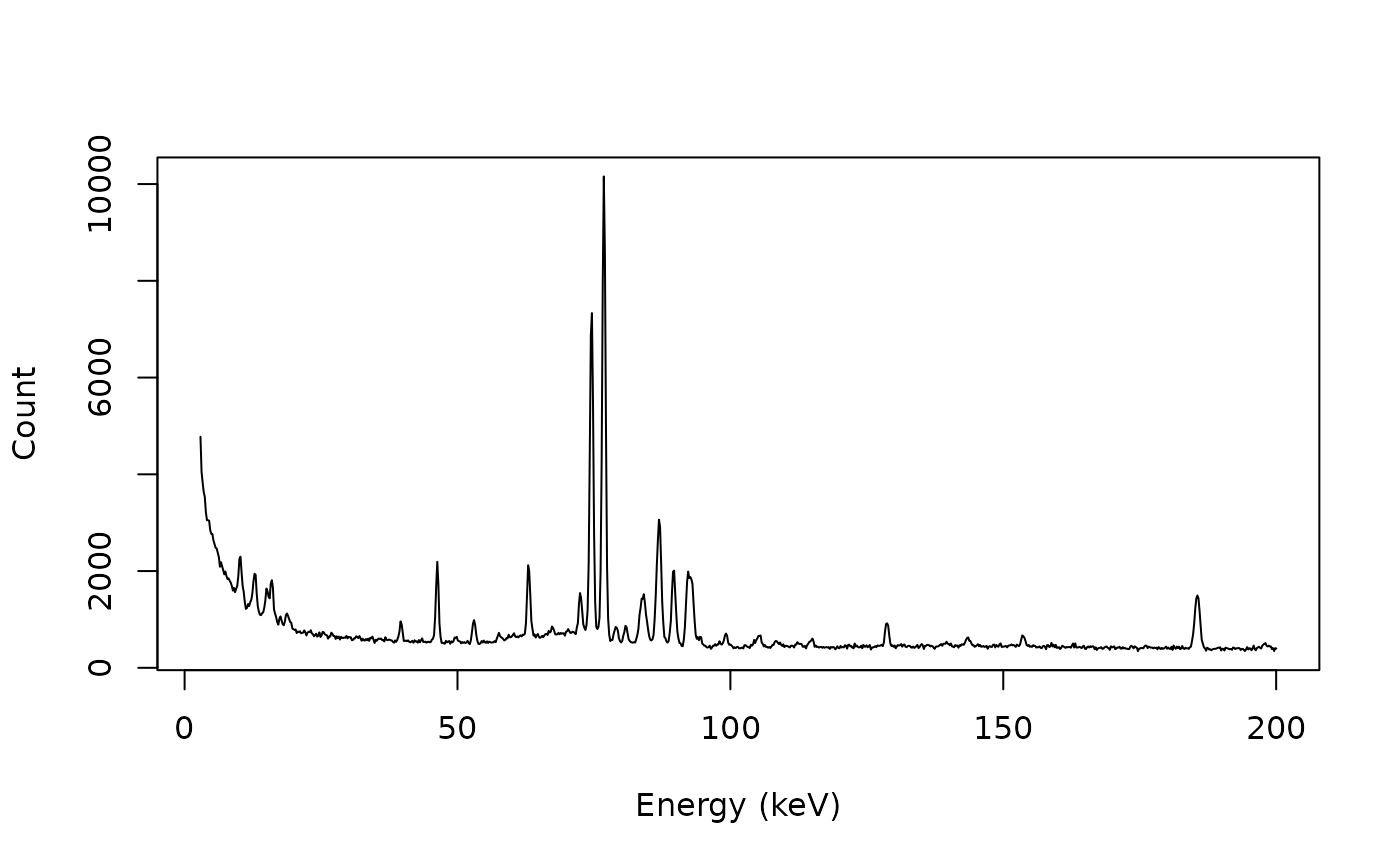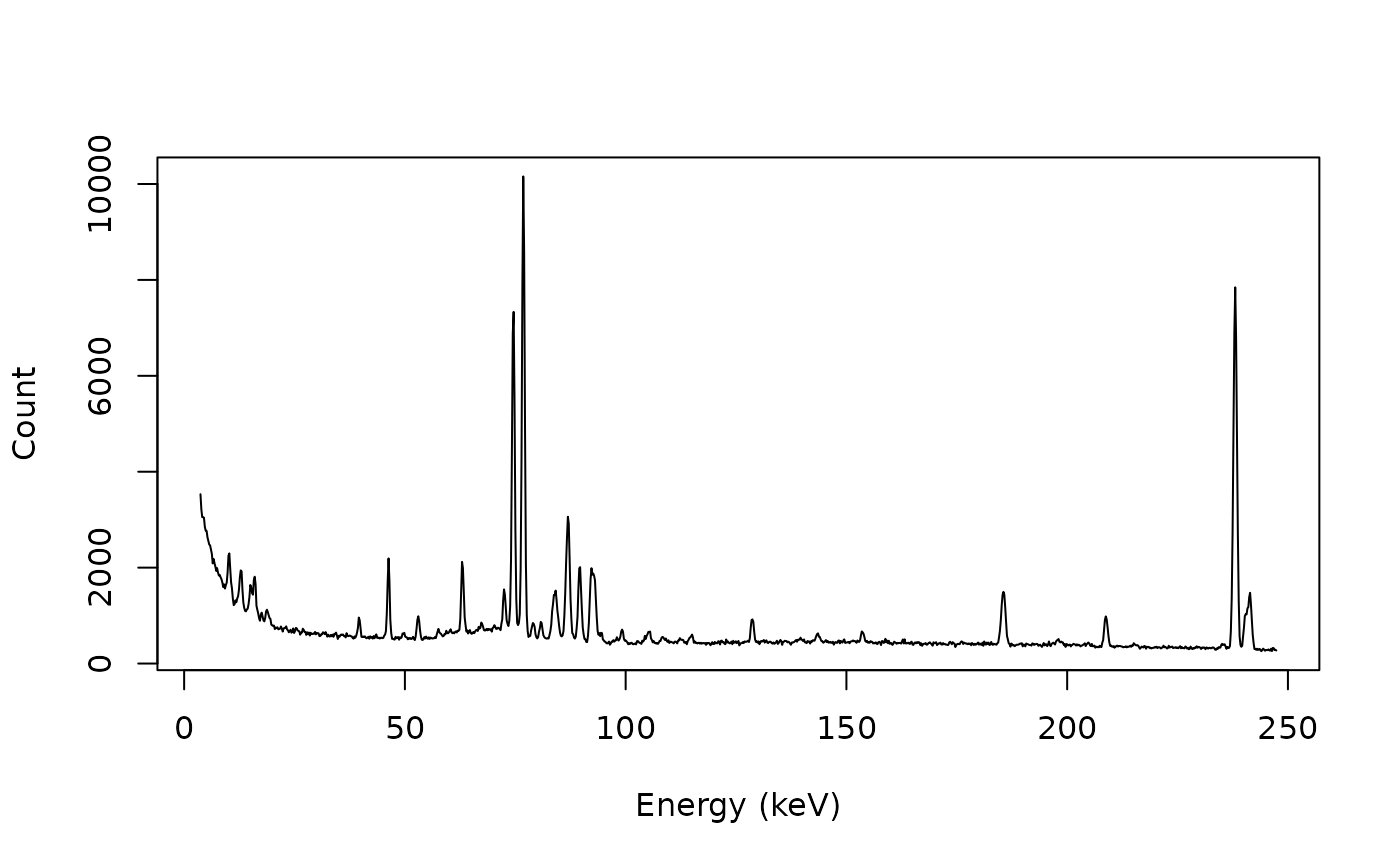• signal_select() allows to subset by values of x.

• signal_slice() allows to subset by position along x.

## Usage

signal_select(x, y, ...)

signal_slice(x, y, ...)

# S4 method for numeric,numeric
signal_select(x, y, from, to)

# S4 method for ANY,missing
signal_select(x, from, to)

# S4 method for numeric,numeric
signal_slice(x, y, subset)

# S4 method for ANY,missing
signal_slice(x, subset)

## Arguments

x, y

A numeric vector. If y is missing, an attempt is made to interpret x in a suitable way (see grDevices::xy.coords()).

...

Currently not used.

from, to

A numeric value giving the first and last value (in x unit) to be selected.

subset

An integer vector giving either positive values to keep, or negative values to drop. The values provided must be either all positive or all negative (coerced to integer as by as.integer()).

## Value

Returns a list with two components x and y.

Other signal processing methods: signal_bind(), signal_correct(), signal_drift(), signal_mean(), signal_shift()

N. Frerebeau

## Examples

## gamma-ray spectrometry
data("BEGe")

## Plot spectrum
plot(BEGe, type = "l", xlab = "Energy (keV)", ylab = "Count")## Subset from 2.75 keV to 200 keV
BEGe_1 <- signal_select(BEGe, from = 3, to = 200)

## Plot spectrum
plot(BEGe_1, type = "l", xlab = "Energy (keV)", ylab = "Count")## Subset from the 20th to the 1250th value
BEGe_2 <- signal_slice(BEGe, subset = 20:1250)

## Plot spectrum
plot(BEGe_2, type = "l", xlab = "Energy (keV)", ylab = "Count")